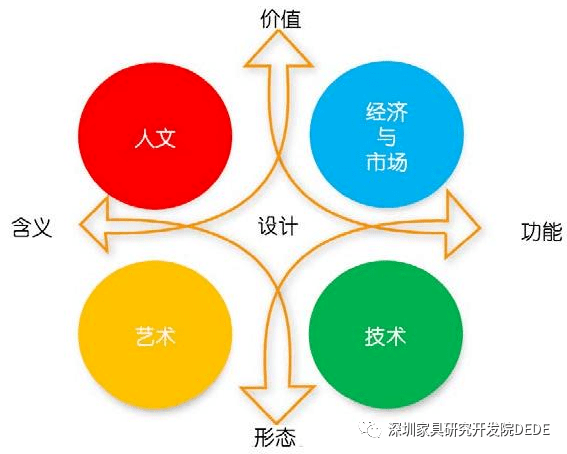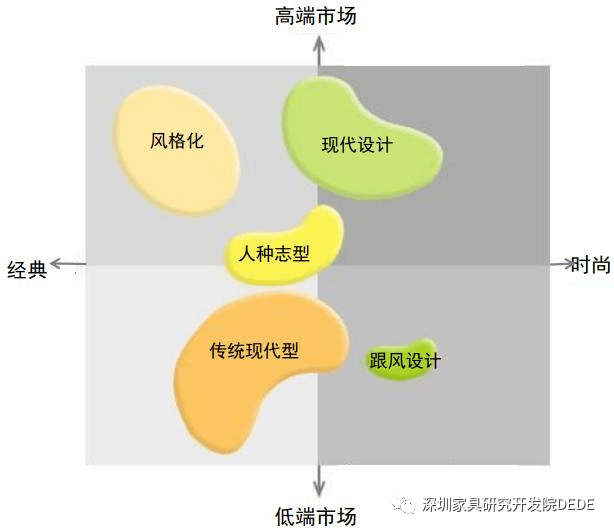|

# 许柏鸣 | 如何围绕家具产品设计创造更大价值?(问答七)1. 市场什么时候才能复苏？依据是什么？

2. 少数头部企业发展势头迅猛，广大中小型企业还有发展的空间和机会吗？如果有，则机会在哪里？

3. 大众红海市场越来越难做，怎么能够在其中活下来？怎么才能发展好？

4. 高端品牌要怎么打造？

5. 如何看待多品牌运行？

6. 如果要避开竞争激烈的红海市场，差异化策略具体要怎么做？

↓本期内容

7. 家具产品设计正在朝什么方向发展？这个方向是否正确，会管多长时间？

8. 错过了跑马圈地的最佳时机，渠道拓展还有机会吗？应该怎么拓展？

9. 房地产行业回暖，低谷期是否已经结束，接下来将如何影响家具行业？

10. 企业人才一直是个大问题，究竟应该怎么解决？John Heskett 2002在《设计简述》中写道：“设计这个词似乎是再普通不过了，然而事实上完全不是这样的，它有数不清的复杂表现，没有清晰的轮廓和边界”。

1）更新对设计的理解

—— 设计，是在行动前先想好要怎么做

—— 设计，是把事情做得更好的途径

—— 设计，是优化客户满意度的手段

—— 设计，是在明确目标导向下的结构化进程

—— 设计，在企业中具有统领作用

—— 设计是探索未来可能性的工具，可称之为“愿景设计”

—— 设计是创新天赋的情感化表达，可称之为“浪漫设计”，设计师是独特的艺术家

—— 设计是产品/服务开发的技术活动，源自需求，可称之为“技术设计”

—— 设计是处于某种境况下的活动统筹，必须清楚其内在规律与交互作用关系并予以协调，可称之为“状态设计”

—— 效率世界，这是指设计所呈现的技术角色，是“设计的工程”范畴

—— 机会世界，这是指设计所呈现的愿景角色，是“设计的进步”范畴2）关于家具产品的设计风格“去风格化”是深圳家具研究开发院对“现代风格”的广谱表述，因为具有两个特点：一是没有明显的区域性标签与符号，二是具有全球意义上的普适性。

3）“风格化”家具的走向

① 原型模仿：遵循历史上古典或传统风格的原貌和原味，只是具体的家具品类需要补充，但依然模仿这种风格的基本形态，因为有些功能古代没有，是由现代生活方式和技术发展催生出来的，如：电视柜、电脑桌和其他电子化与数字化工作、生活与娱乐设施所用到的家具，新的家具品类还会继续随着新的生活方式的出现而出现，如一些新的智能化家具等；

② 原型变异：原型变异与复制延展没有明显的分界线，而是无级变化的。如欧洲新古典主义风格中，车腿与八角型台面深具特色，抓住了这两个特征，那么就可以演绎出无数新的家具形态；

③ 原型重构：重构方式是汲取古典风格家具中具有视觉效力的一种或多种特性来重新进行设计，这些特征或特质可以是形态方面的，也可以是装饰元素上的，还可以是CMF以及这些手法的混合应用上的。目前国内市场上很多“新中式”家具就属于这个范畴；

④ 全新设计：这是从具象切换为抽象的设计方法，所追求的不再是借鉴古典风格家具中的具象元素，而是取其神韵，对设计师的功力要求极高，因为更加没有规律、难以驾驭，需要具备更高的设计、材料、工艺、技术、艺术和人文方面的造诣，乃至哲学素养。

“软现代”是现代与古典或传统的一种对话与纽带，“根系现代、魂归传统”。

4）“去风格化”家具的设计走向

5） 围绕产品创造更大价值

“伟大的创造力不是要改造车轮，而是改造汽车”，设计师们变得更具战略意义，因为他们正在向食物链的高端移动。

① 要从小设计向大设计方向发展，即原先设计只是生产系统的附属，样品完成后设计的任务基本完成；但现在与未来，设计还要延伸到市场、分销、递送、消费，乃至产品生命周期结束后的处置等所有环节；

② 家具产品的设计要与空间外环境相融合，即要走向一体化设计。但一体化设计并不是说，对每一个室内外空间都需要量身定制每一件家具产品，而是必须建立在标准化平台基础上的柔性输出，不是强制绑定，而是“松耦合(SOA）”

③ 设计要从单纯设计款式切换为产品全要素设计，并上升到企业战略和文化层面；

④ 产品风格有两大类，一是古典与传统上的风格化家具，二是去风格化的现代与当代家具。但企业和品牌要依据自身的基因、独特资源和能力做出不同的特色、要有差异化；同时，还要随着潮流趋势的变化而变化；

⑤ 围绕产品创造更大价值。

`声明：本文由入驻焦点开放平台的作者撰写，除焦点官方账号外，观点仅代表作者本人，不代表焦点立场错误信息举报电话： 400-099-0099，邮箱：jubao@vip.sohu.com，或点此进行意见反馈，或点此进行举报投诉。`

A B C D E F G H J K L M N P Q R S T W X Y Z
A - B - C - D - E
• A
• 鞍山
• 安庆
• 安阳
• 安顺
• 安康
• 澳门
• B
• 北京
• 保定
• 包头
• 巴彦淖尔
• 本溪
• 蚌埠
• 亳州
• 滨州
• 北海
• 百色
• 巴中
• 毕节
• 保山
• 宝鸡
• 白银
• 巴州
• C
• 承德
• 沧州
• 长治
• 赤峰
• 朝阳
• 长春
• 常州
• 滁州
• 池州
• 长沙
• 常德
• 郴州
• 潮州
• 崇左
• 重庆
• 成都
• 楚雄
• 昌都
• 慈溪
• 常熟
• D
• 大同
• 大连
• 丹东
• 大庆
• 东营
• 德州
• 东莞
• 德阳
• 达州
• 大理
• 德宏
• 定西
• 儋州
• 东平
• E
• 鄂尔多斯
• 鄂州
• 恩施
F - G - H - I - J
• F
• 抚顺
• 阜新
• 阜阳
• 福州
• 抚州
• 佛山
• 防城港
• G
• 赣州
• 广州
• 桂林
• 贵港
• 广元
• 广安
• 贵阳
• 固原
• H
• 邯郸
• 衡水
• 呼和浩特
• 呼伦贝尔
• 葫芦岛
• 哈尔滨
• 黑河
• 淮安
• 杭州
• 湖州
• 合肥
• 淮南
• 淮北
• 黄山
• 菏泽
• 鹤壁
• 黄石
• 黄冈
• 衡阳
• 怀化
• 惠州
• 河源
• 贺州
• 河池
• 海口
• 红河
• 汉中
• 海东
• 怀来
• I
• J
• 晋中
• 锦州
• 吉林
• 鸡西
• 佳木斯
• 嘉兴
• 金华
• 景德镇
• 九江
• 吉安
• 济南
• 济宁
• 焦作
• 荆门
• 荆州
• 江门
• 揭阳
• 金昌
• 酒泉
• 嘉峪关
K - L - M - N - P
• K
• 开封
• 昆明
• 昆山
• L
• 廊坊
• 临汾
• 辽阳
• 连云港
• 丽水
• 六安
• 龙岩
• 莱芜
• 临沂
• 聊城
• 洛阳
• 漯河
• 娄底
• 柳州
• 来宾
• 泸州
• 乐山
• 六盘水
• 丽江
• 临沧
• 拉萨
• 林芝
• 兰州
• 陇南
• M
• 牡丹江
• 马鞍山
• 茂名
• 梅州
• 绵阳
• 眉山
• N
• 南京
• 南通
• 宁波
• 南平
• 宁德
• 南昌
• 南阳
• 南宁
• 内江
• 南充
• P
• 盘锦
• 莆田
• 平顶山
• 濮阳
• 攀枝花
• 普洱
• 平凉
Q - R - S - T - W
• Q
• 秦皇岛
• 齐齐哈尔
• 衢州
• 泉州
• 青岛
• 清远
• 钦州
• 黔南
• 曲靖
• 庆阳
• R
• 日照
• 日喀则
• S
• 石家庄
• 沈阳
• 双鸭山
• 绥化
• 上海
• 苏州
• 宿迁
• 绍兴
• 宿州
• 三明
• 上饶
• 三门峡
• 商丘
• 十堰
• 随州
• 邵阳
• 韶关
• 深圳
• 汕头
• 汕尾
• 三亚
• 三沙
• 遂宁
• 山南
• 商洛
• 石嘴山
• T
• 天津
• 唐山
• 太原
• 通辽
• 铁岭
• 泰州
• 台州
• 铜陵
• 泰安
• 铜仁
• 铜川
• 天水
• 天门
• W
• 乌海
• 乌兰察布
• 无锡
• 温州
• 芜湖
• 潍坊
• 威海
• 武汉
• 梧州
• 渭南
• 武威
• 吴忠
• 乌鲁木齐
X - Y - Z
• X
• 邢台
• 徐州
• 宣城
• 厦门
• 新乡
• 许昌
• 信阳
• 襄阳
• 孝感
• 咸宁
• 湘潭
• 湘西
• 西双版纳
• 西安
• 咸阳
• 西宁
• 仙桃
• 西昌
• Y
• 运城
• 营口
• 盐城
• 扬州
• 鹰潭
• 宜春
• 烟台
• 宜昌
• 岳阳
• 益阳
• 永州
• 阳江
• 云浮
• 玉林
• 宜宾
• 雅安
• 玉溪
• 延安
• 榆林
• 银川
• Z
• 张家口
• 镇江
• 舟山
• 漳州
• 淄博
• 枣庄
• 郑州
• 周口
• 驻马店
• 株洲
• 张家界
• 珠海
• 湛江
• 肇庆
• 中山
• 自贡
• 资阳
• 遵义
• 昭通
• 张掖
• 中卫

1室1厅1厨1卫1阳台

1
2
3
4
5

0
1
2

1

1

0
1
2
3报名成功，资料已提交审核A B C D E F G H J K L M N P Q R S T W X Y Z
A - B - C - D - E
• A
• 鞍山
• 安庆
• 安阳
• 安顺
• 安康
• 澳门
• B
• 北京
• 保定
• 包头
• 巴彦淖尔
• 本溪
• 蚌埠
• 亳州
• 滨州
• 北海
• 百色
• 巴中
• 毕节
• 保山
• 宝鸡
• 白银
• 巴州
• C
• 承德
• 沧州
• 长治
• 赤峰
• 朝阳
• 长春
• 常州
• 滁州
• 池州
• 长沙
• 常德
• 郴州
• 潮州
• 崇左
• 重庆
• 成都
• 楚雄
• 昌都
• 慈溪
• 常熟
• D
• 大同
• 大连
• 丹东
• 大庆
• 东营
• 德州
• 东莞
• 德阳
• 达州
• 大理
• 德宏
• 定西
• 儋州
• 东平
• E
• 鄂尔多斯
• 鄂州
• 恩施
F - G - H - I - J
• F
• 抚顺
• 阜新
• 阜阳
• 福州
• 抚州
• 佛山
• 防城港
• G
• 赣州
• 广州
• 桂林
• 贵港
• 广元
• 广安
• 贵阳
• 固原
• H
• 邯郸
• 衡水
• 呼和浩特
• 呼伦贝尔
• 葫芦岛
• 哈尔滨
• 黑河
• 淮安
• 杭州
• 湖州
• 合肥
• 淮南
• 淮北
• 黄山
• 菏泽
• 鹤壁
• 黄石
• 黄冈
• 衡阳
• 怀化
• 惠州
• 河源
• 贺州
• 河池
• 海口
• 红河
• 汉中
• 海东
• 怀来
• I
• J
• 晋中
• 锦州
• 吉林
• 鸡西
• 佳木斯
• 嘉兴
• 金华
• 景德镇
• 九江
• 吉安
• 济南
• 济宁
• 焦作
• 荆门
• 荆州
• 江门
• 揭阳
• 金昌
• 酒泉
• 嘉峪关
K - L - M - N - P
• K
• 开封
• 昆明
• 昆山
• L
• 廊坊
• 临汾
• 辽阳
• 连云港
• 丽水
• 六安
• 龙岩
• 莱芜
• 临沂
• 聊城
• 洛阳
• 漯河
• 娄底
• 柳州
• 来宾
• 泸州
• 乐山
• 六盘水
• 丽江
• 临沧
• 拉萨
• 林芝
• 兰州
• 陇南
• M
• 牡丹江
• 马鞍山
• 茂名
• 梅州
• 绵阳
• 眉山
• N
• 南京
• 南通
• 宁波
• 南平
• 宁德
• 南昌
• 南阳
• 南宁
• 内江
• 南充
• P
• 盘锦
• 莆田
• 平顶山
• 濮阳
• 攀枝花
• 普洱
• 平凉
Q - R - S - T - W
• Q
• 秦皇岛
• 齐齐哈尔
• 衢州
• 泉州
• 青岛
• 清远
• 钦州
• 黔南
• 曲靖
• 庆阳
• R
• 日照
• 日喀则
• S
• 石家庄
• 沈阳
• 双鸭山
• 绥化
• 上海
• 苏州
• 宿迁
• 绍兴
• 宿州
• 三明
• 上饶
• 三门峡
• 商丘
• 十堰
• 随州
• 邵阳
• 韶关
• 深圳
• 汕头
• 汕尾
• 三亚
• 三沙
• 遂宁
• 山南
• 商洛
• 石嘴山
• T
• 天津
• 唐山
• 太原
• 通辽
• 铁岭
• 泰州
• 台州
• 铜陵
• 泰安
• 铜仁
• 铜川
• 天水
• 天门
• W
• 乌海
• 乌兰察布
• 无锡
• 温州
• 芜湖
• 潍坊
• 威海
• 武汉
• 梧州
• 渭南
• 武威
• 吴忠
• 乌鲁木齐
X - Y - Z
• X
• 邢台
• 徐州
• 宣城
• 厦门
• 新乡
• 许昌
• 信阳
• 襄阳
• 孝感
• 咸宁
• 湘潭
• 湘西
• 西双版纳
• 西安
• 咸阳
• 西宁
• 仙桃
• 西昌
• Y
• 运城
• 营口
• 盐城
• 扬州
• 鹰潭
• 宜春
• 烟台
• 宜昌
• 岳阳
• 益阳
• 永州
• 阳江
• 云浮
• 玉林
• 宜宾
• 雅安
• 玉溪
• 延安
• 榆林
• 银川
• Z
• 张家口
• 镇江
• 舟山
• 漳州
• 淄博
• 枣庄
• 郑州
• 周口
• 驻马店
• 株洲
• 张家界
• 珠海
• 湛江
• 肇庆
• 中山
• 自贡
• 资阳
• 遵义
• 昭通
• 张掖
• 中卫• 手机• 分享
• 设计
免费设计
• 计算器
装修计算器
• 联系
• 置顶
返回顶部